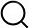# Also in the Article

Statistical analysis: Exact grid enumeration

Procedure

We began our analysis by enumerating all grid diagrams with grid number 5 ≤ GN ≤ 7. This was achieved using a Sage (34) program by listing all possible pairs of permutations of length 5 ≤ n ≤ 7 and keeping the ones representing knot diagrams. The topological state (i.e., the underlying knot type) of every configuration was determined using a combination of knot invariants [knot polynomials, determinant, and signature (24)]. We then performed every possible crossing change from each configuration. We summarized the data in a square table, the unbiased adjacency table, in which the (i,j)-th entry represents the number of strand passages from the i-th knot type to the j-th knot type. The adjacency table was then visualized using circos plots (see Fig. 3) (35). The previous process was then repeated, this time, by allowing only strand passages to occur at hooked juxtapositions (see Fig. 2). While the unbiased adjacency table is symmetric, the hooked one presents a strong unknotting preference, as discussed in Results.

Q&A